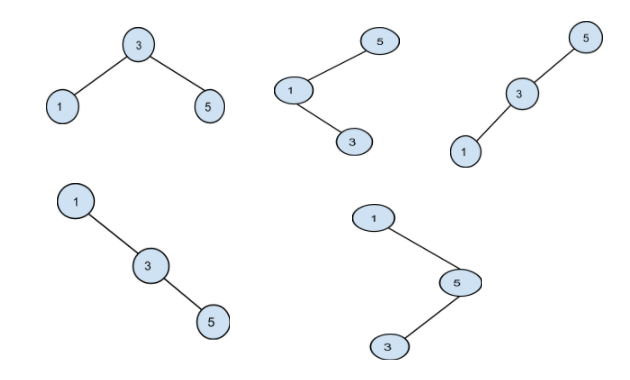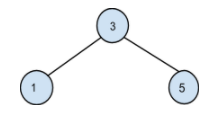5

# Optimal BST

Difficulty: HARD
Avg. time to solve
55 min
Success Rate
45%

Problem Statement
Suggest Edit

#### Given a sorted array of keys of BST and an array of frequency counts of each key in the same order as in the given inorder traversal. The frequency of an element is the number of searches made to that element. Construct a binary search tree from the given keys such that the total cost of all the searches is minimum.

``````Cost of searching a key is its frequency multiplied by its level number in the BST.
``````

#### For example:

``````Input keys = [ 1, 3, 5 ]
Frequency = [ 3, 10, 7 ]

All the unique BST possible from the given keys are:
````````````Among all these possible BST, the minimum cost is obtained from the below BST:
````````````Cost = 1 * (freq of 3) + 2 * (freq of 1) + 2 * (freq of 5) = 30
where 1 is the level of node 3, 2 is the level of the node 1 and node 5.
``````
##### Input Format
``````The first line of input contains an integer T, the number of test cases.
The first line of each test case contains an element ‘N’ denoting the number of elements in the BST.
The second line of each test case contains ‘N’ space separated integers, sorted in ascending order.
The third line of each test case contains ‘N’ space separated integers denoting the frequency of each element of the BST.
``````
##### Output Format:
``````For every test case, print the minimum total cost of constructing the BST.
The output of each test case is printed in a separate line.
``````
##### Note
``````1. Given BST will not contain duplicates.
2. You don’t have to print anything, it has already been taken care of. Just implement the function.
``````
##### Constraints:
``````1 <= T <= 5
2 <= N <= 50
0 <= data <= 10^4

Where ‘T’ is the number of test cases, ‘N’ is the number of nodes in the given tree and ‘data’ denotes the value of the nodes in the given tree.
``````
##### Sample Input 1:
``````1
3
1 3 5
3 10 7
``````
##### Sample Output 1:
``````30
``````
##### Explanation of sample input 1:
``````All the possible BST’s for the given input are shown in the above example.

Searching cost in first BST = 1 * 10 + 2 * 3 + 2 * 7 = 30.
Searching cost in second BST = 1 * 7 + 2 * 3 + 3 * 10 = 43.
Searching cost in third BST = 1 * 7 + 2 * 10 + 3 * 3 = 36.
Searching cost in fourth BST = 1 * 3 + 2 * 10 + 3 * 7 = 44.
Searching cost in fifth BST = 1 * 3 + 2 * 7 + 3 * 10 = 47.

Thus, 30 is the minimum cost of all the searches.
``````
##### Sample Input 2:
``````1
3
1 2 3
25 10 20
``````
##### Sample Output 2
``````95
``````Console